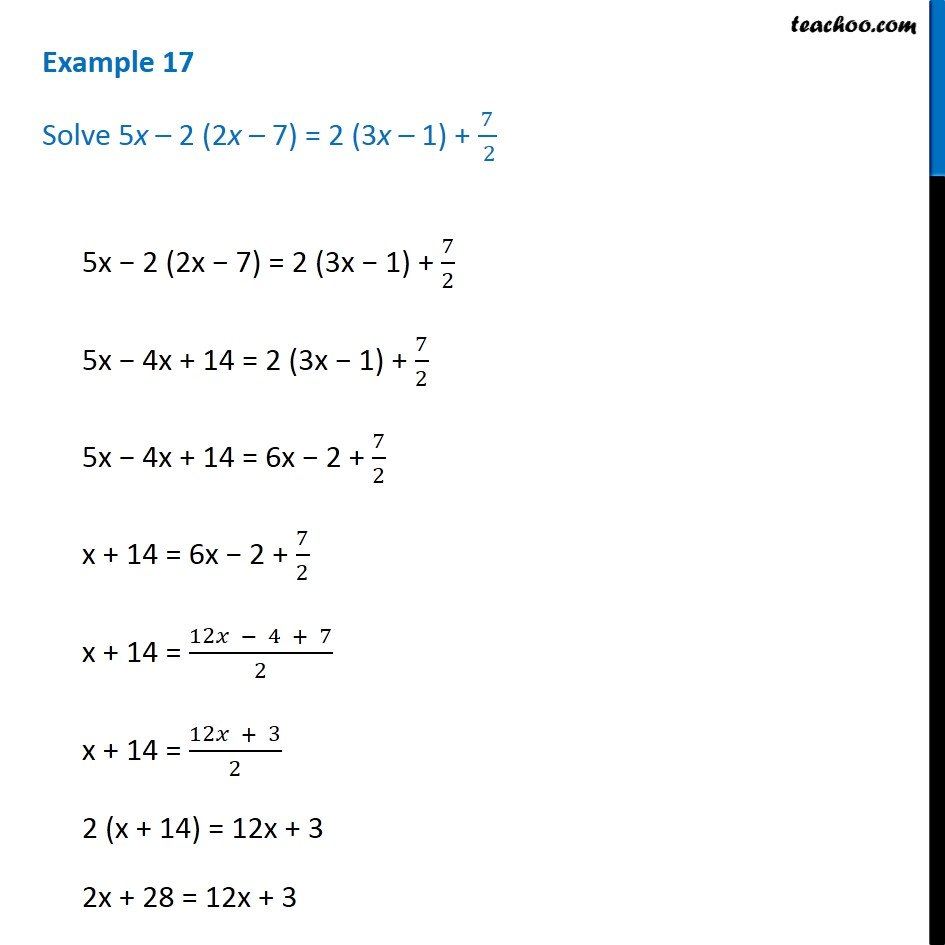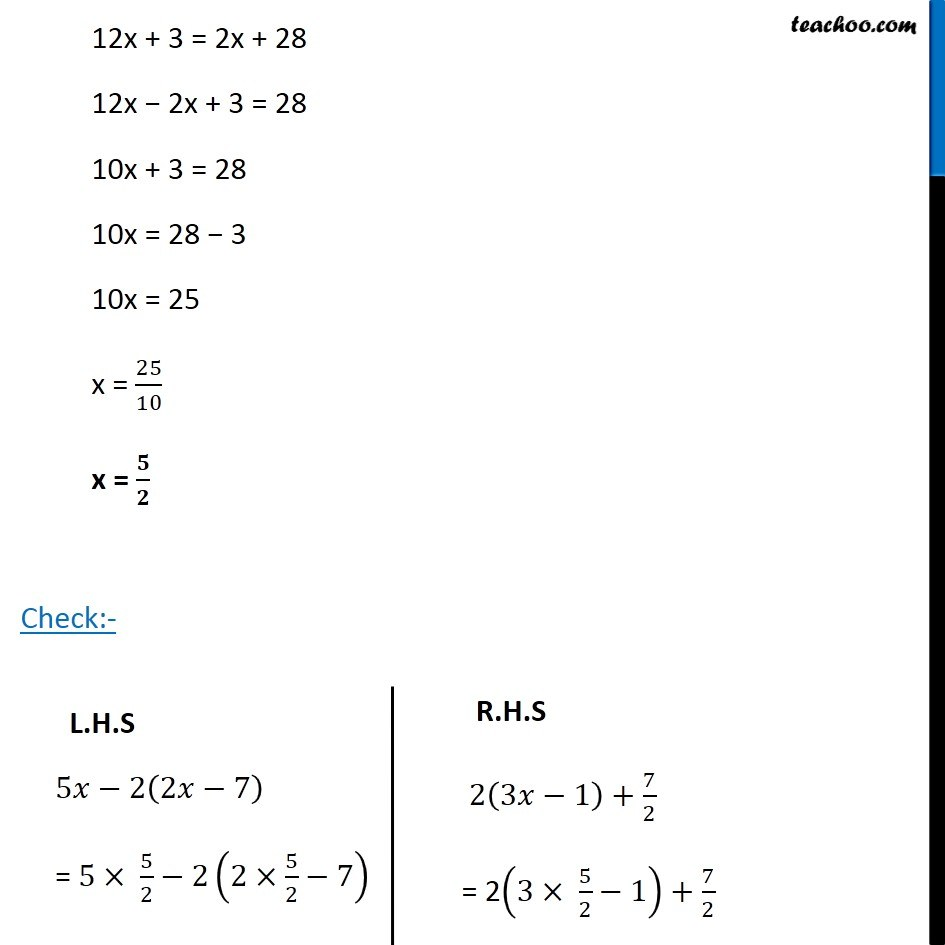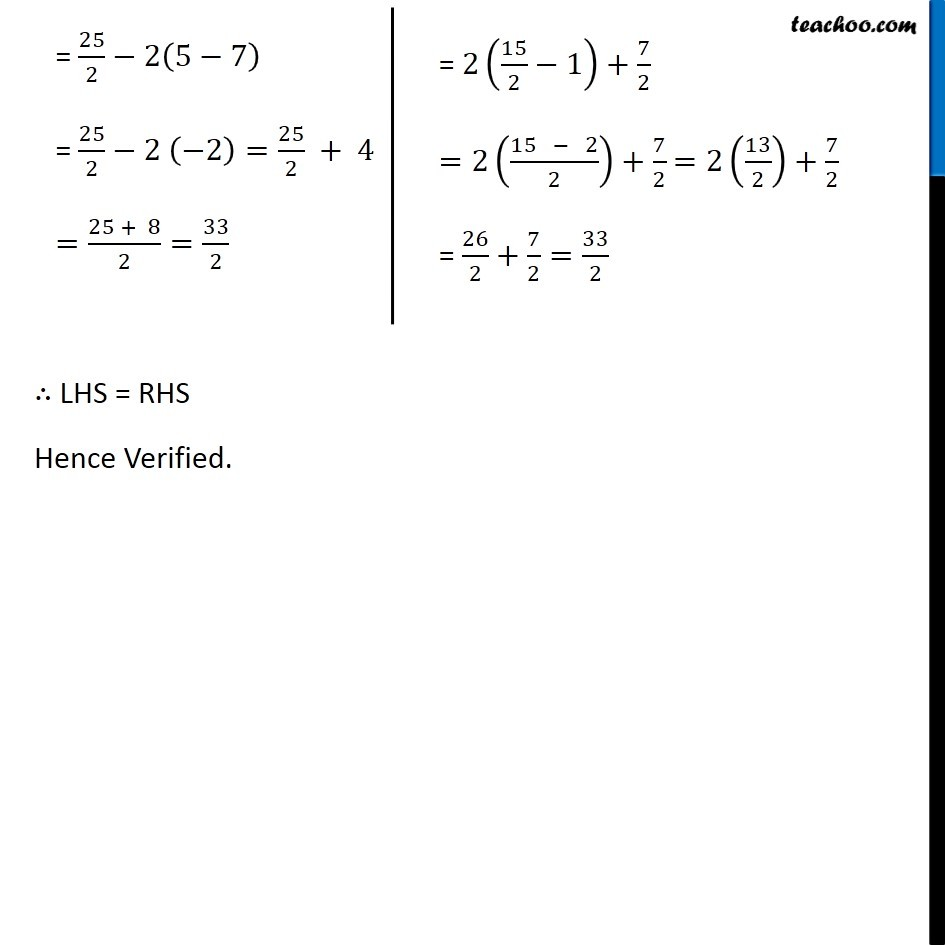1. Chapter 2 Class 8 Linear Equations in One Variable
2. Serial order wise
3. Examples

Transcript

Example 17 Solve 5x – 2 (2x – 7) = 2 (3x – 1) + 7/( 2)5x − 2 (2x − 7) = 2 (3x − 1) + 7/2 5x − 4x + 14 = 2 (3x − 1) + 7/2 5x − 4x + 14 = 6x − 2 + 7/2 x + 14 = 6x − 2 + 7/2 x + 14 = (12𝑥 − 4 + 7)/2 x + 14 = (12𝑥 + 3)/2 2 (x + 14) = 12x + 3 2x + 28 = 12x + 3 12x + 3 = 2x + 28 12x − 2x + 3 = 28 10x + 3 = 28 10x = 28 − 3 10x = 25 x = 25/10 x = 𝟓/𝟐 Check:- L.H.S 5𝑥−2(2𝑥−7) = 5× 5/2−2(2×5/2−7) R.H.S 2(3𝑥−1)+7/2 = 2(3× 5/2−1)+7/2 = 25/2−2(5−7) = 25/2−2 (−2)=25/2 + 4 =(25 + 8)/2=33/2 = 2(15/2−1)+7/2 =2((15 − 2)/2)+7/2 =2(13/2)+7/2 = 26/2+7/2=33/2 ∴ LHS = RHS Hence Verified.

Examples